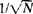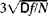# Statistical Simulation

## Statistical Simulation

a numerical method of solving mathematical problems. In this method, the desired quantities are represented as probabilistic characteristics of some random phenomenon, a mathematical model of the phenomenon is constructed, and the needed quantities are approximately determined by statistical analysis of “observations” on the model.

Suppose, for example, we wish to calculate the heat flux in a heated thin metal plate at whose edges a zero temperature is maintained. The heat distribution is described by the same equation as the spreading of a spot of dye in a layer of liquid. The planar Brownian motion of the “dye” particles on the plate is therefore modeled by tracking their positions at times , where k = 0, 1, 2, .... It is assumed on an approximate basis that in the short interval τ a particle moves a distance of h with equal probability in all directions. Each time the direction is selected randomly, without regard to the preceding motion. The relation between τ and h is determined by the thermal conductivity. The motion begins at the heat source and ends when an edge is first reached (when sticking of the “dye” to the edge is observed). The heat flow Q(C) across a section C of the boundary is measured by the amount of adhering dye. When the total number of particles is N, such an estimate has, according to the law of large numbers, a random relative error of the order ofthe estimate has a systematic error of the order of h owing to the discreteness of the selected model.

The desired quantity is represented by the mathematical expectation of a numerical function f of the random outcome ѡ of the phenomenon: Ef(ѡ) = ʃ(ѡ) dP. In other words, the desired quantity is represented by the integral with respect to the probability measure P (seeMEASURE OF A SET). The estimator Ef(ѡ) ≈ [f(ѡ1) + ... + f(ѡN)]/N, where ѡ1, . . ., ѡNare the simulated outcomes, can be regarded as the quadrature formula for the indicated integral with random nodes ѡk and random error RN. Usually ǀRNǀ is considered to be less than or equal toon the assumption that a large error is of negligible probability; the variance Df can be estimated in the course of the observations (seeERRORS, THEORY OF).

In the example discussed above, f(ѡ) = 1 when the path ends at C; otherwise, f(ѡ) = 0. The variance Df = [1 – Q(C)]Q(C) ≤ 1/4. The integral is taken over a space of polygonal lines with segments of constant length and can be evaluated by means of multiple integrals.

Each “experiment” is carried out in two steps: the “drawing” of a random outcome a> and the subsequent calculation of the function f(ѡ). When the space of all outcomes and the probability measure P are too complicated, the draw is made successively in several stages, as in the example above. A random selection is made in each stage by means of random numbers, such as the numbers produced by a random number generator. Pseudorandom numbers, which represent an arithmetic imitation of random numbers, may also be used (seeRANDOM AND PSEUDORANDOM NUMBERS). Similar procedures of random selection are used in mathematical statistics and game theory.

Statistical simulation is widely used for the computer solution of integral equations—for example, in the investigation of complex systems. The method is convenient in its universality and generally does not require a large memory. A shortcoming is the number of large random errors, which decrease extremely slowly as the number of experiments increases. For this reason, methods of model transformation that make it possible to reduce the spread of the observed quantities and the size of the modeling experiment have been worked out.

### REFERENCES

Metod statistkheskikh ispytanii (Metod Monte-Karlo). Moscow, 1962.
Ermakov, S. M. Metod Monte-Karlo i smezhnye voprosy. Moscow, 1971.

N. N. CHENTSOV

Mentioned in ?
References in periodicals archive ?
This study supplements our recent findings by investigating through statistical simulation how the injury risk varies if a large number of experimental tests were conducted.
Among the topics are probability, random number generation, the statistical simulation of the linear model, testing theory using simulation, and other simulation-based methods.
Research published in Physical Review Letters shows that a technique called direct statistical simulation accurately models fluid jets, fast-moving flows that form naturally in oceans and in the atmosphere.
It includes innovative algorithms and parallel technologies to accelerate statistical simulation performance.
As such, the game can be read as an under-sketching of the statistical simulation of existence that looks back over the history of this key tradition of governmental and instrumental control, while proposing its continuity in the pedagogical practices of the new media age.
Numerical methods that are known as Monte Carlo methods can be loosely described as statistical simulation methods, where statistical simulation is defined to be any method that utilizes sequences of random numbers to perform the simulation.
They present chapters covering basic concepts of vector space theory of matter, consequences of quantum mechanics, chemistry and quantum mechanics, approximations for many-electron systems, perturbation theory in quantum chemistry, group theory in theoretical chemistry, computational quantum chemistry methods, force field methods and molecular modeling, and Monte Carlo and molecular dynamics methods of statistical simulation.
In this research a chemical mass balance model with Monte Carlo statistical simulation using Microsoft Visual Basic 6.
The topics include a high-performance tool for testing long memory and self-similarity, an accurate and reliable simulation framework for mobile computing systems using stochastic differential equations, the statistical simulation of multi-path fading channels for mobile wireless digital communication systems, comparing non-probing-based routing metrics for static multi-hop underwater acoustic networks, and simulating communications and networking in vehicular ad hoc networks.
In addition, if noise figure performance of the device was modeled by on-wafer measurements, then a FET model with built-in noise parameter support such as MMICAD's FETN can be used to provide statistical simulation of noise figure as well.

Site: Follow: Share:
Open / Close